# High Performance JavaScript

August 16,2020☕️ 5 min read

In JavaScript, we can do the same thing in more than one way and its a good thing(mostly!)., even though some ways are not recommended, JavaScript has it. The only reason for such parts in JavaScript is, fixing it will break all those web application built using those bad parts.

In this post we are going to see the best and highly efficient methods for some popular and most used methods. We are not going to see how each method works instead we will be running performance tests and finding the high performing method.

### Converting String to Number

As JavaScript is dynamically typed, type conversion is one of the most used feature in almost all applications. We have more than one method to convert a string to number.

const str = "100";
let num;
num = parseInt(str,10);
num = parseFloat(str);
num = str << 0;
num = +str;
num = str*1;
num = str-0;
num = Number(str);

parseInt is faster than other ways and I have run the performance test on these methods.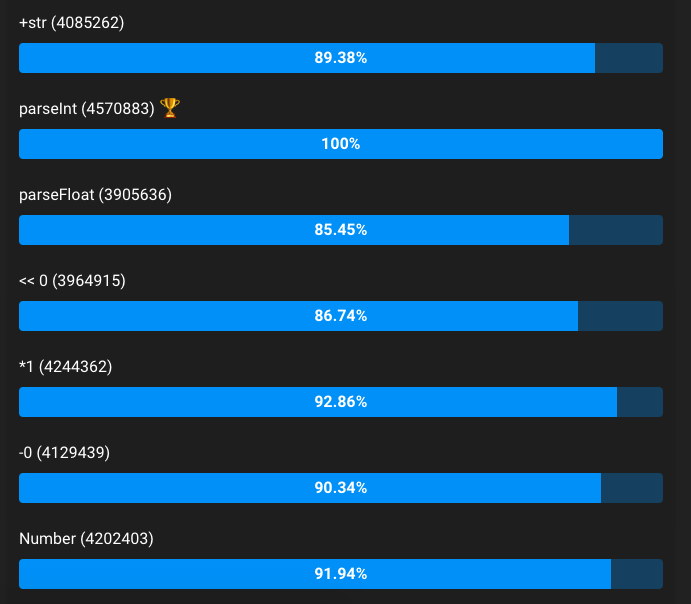#### Why use parseInt apart from performance reasons?

parseInt detects a leading zero on the string, it will parse the number in octal base, this has changed on ECMAScript 5, the new version of the standard, but it will take a long time to get in browser implementations (it’s an incompatibility with ECMAScript 3), also parseInt will ignore trailing characters that don’t correspond with any digit of the currently used base.

parseInt(“20px”);       // 20
parseInt(“10100”, 2);   // 20
parseInt(“2e1”);        // 2

We can also pass radix and define octal value in parseInt

parseInt(“010”);       // 8, implicit octal
parseInt(“010”, 10);   // 10, decimal radix

### Fastest way to iterate through for loop

Next to data type conversion, we will use a for loop in many scenarios and as always JavaScript provides more that one way to iterate through the data.

// const arr = [...];
// let assign;
//plain loop
for (let x = 0; x < arr.length; x++) {
assign = arr[x];
}
//plain loop with cached length
const len = arr.length;
for (let x = 0; x < len; x++) {
assign = arr[x];
}
//for-of loop
for (let val of arr) {
assign = val;
}
//forEach
arr.forEach(function(value, index) {
assign = value;
});
//i-- plain loop
for (var x = arr.length; x >= 0; x--) {
assign = arr[x];
}
//i-- plain loop with cached length
var len = arr.length;
for (var x = len; x >= 0; x--) {
assign = arr[x];
}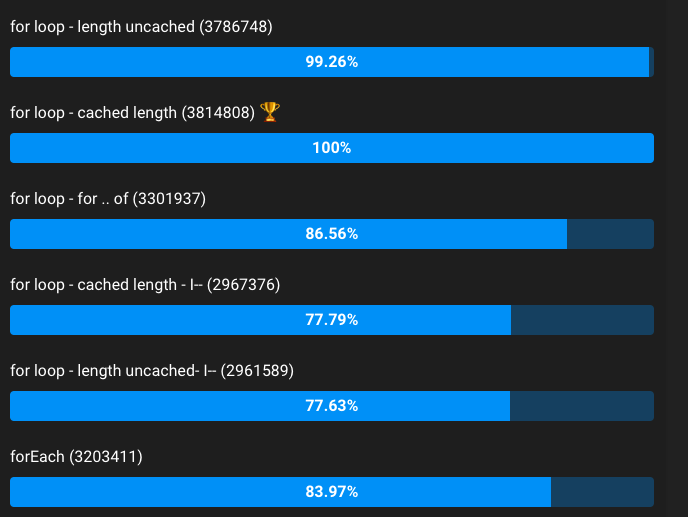As we can see, The fastest loop is plain for loop, both with and without caching length delivering really similar performance. The for loop with cached length sometimes delivered better results than the one without caching, but the difference is almost negligible.

### String Concatenation

We can concat a string by using += or array.join() but which is faster?

// +=
let result = "";
for (let i = 0; i < 1e6; i++) {
result += "test";
}
//array.join
let array = new Array(1e6);
for (let i = 0; i < 1e6; i++) {
array[i] = "test";
}
result = array.join("");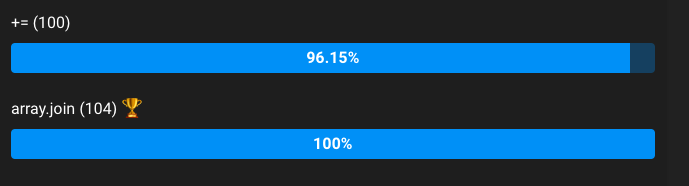As you can see, array.join is providing better performance that Normal String concatenation. With respect to String Concatenation, we can use array.join.

### array.push vs array[length]

When building a fairly large application in JavaScript, we tend to use arrays. And again we have more than one ways to insert the data into an array. We will be doing performance tests for top two ways to insert data in an array.

//array.push
let result = [];
for (let i=0;i<2e6;i++){
result.push(i);
}
//array[n]
let result = [];
for (let i=0;i<2e6;i++){
result[i] = i;
}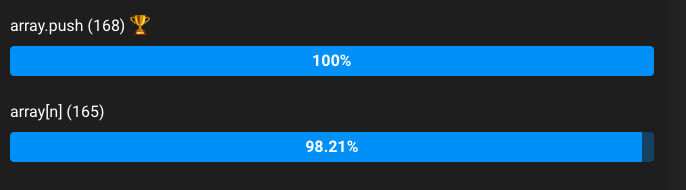Again there is not much of a difference between array.push and array[n], Personally I prefer accessing using length than pushing because we know exactly where we’re adding the data.

### Find the Number of Keys in an Object

JSON is used in all applications and one common task for Web based application is to find the key size, so we can effectively use a graph or chart or table to visualise it.

//Object.keys
//var obj = {...};
var len = 0;
len = Object.keys(obj).length;
//for loop
var len = 0;
for(var prop in obj) {
if(obj.hasOwnProperty(prop))
++len;
}
//lodash
var len = 0;
len = _.size(obj);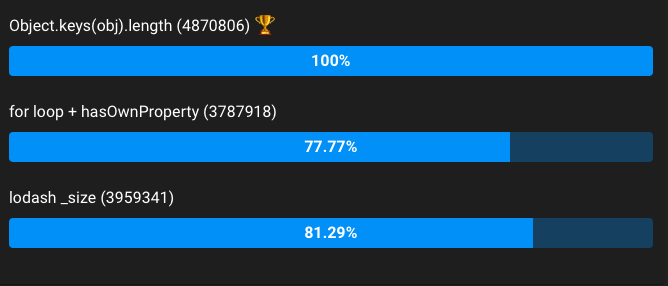Object.keys(obj).length; Works by iterating over the keys to compute a temporary array and returns its length. Its more readable and clean syntax. Plus we don’t need a library or custom code required except a shim if native support is unavailable.

Thanks for reading! Yes, we skipped some other ways which we can use to perform these operations, But I hope that you had a better insight on performance metrics of these operations than before.

Lets build a faster web.